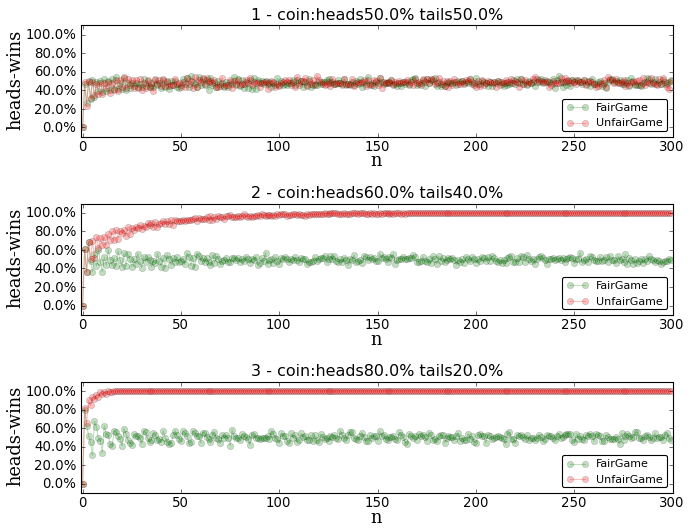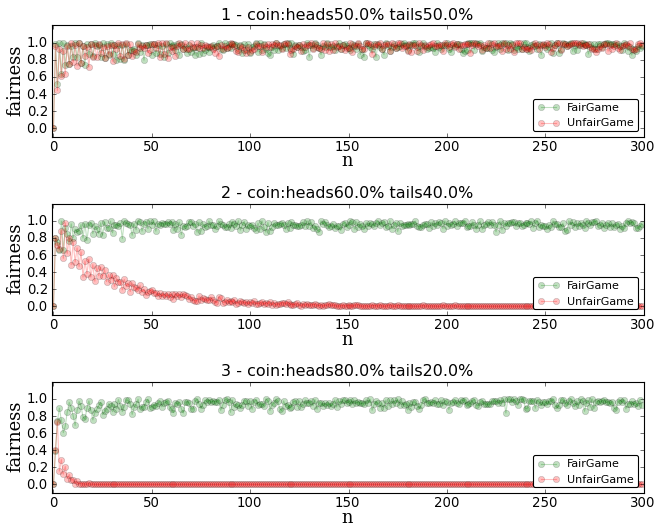November 20, 2013

# Getting a fair result with an unfair coin

How can you get a fair coin toss if someone hands you a coin that is weighted to come up heads more often than tails?

### Solution

This is @luismartingil solution. Please check all the Python source code here. This solution uses `matplotlib` for the plots.

• We can have two different types of results when throwing the coin: {heads, tails}

• We are going to have two players playing the game: {`player-heads`, `player-tails`}
• `player-heads` is the player that chooses heads. `player-tails` the one choosing tails.
• A fair game means that both players have the same probability of winning (50%).

We first need to know how unfair the game is in order to make it fair. We have to know how many times the coin comes up heads vs the times it comes up tails. We do this throwing the coin N times, where N is a huge number. After doing this we have a percentage of the times the coin comes up heads, lets call it `p1`. We can define the percentage of times the coin comes up tails as `p2`.

Based on the problem: - `p1` and `p2` are in this range `[0, 100]` - `p1` will be bigger than `p2`. `p1 > p2`

We can assume that `p2 = 100 - p1`.

In order the game to be fair, `player-heads` has to win more than the `p1` times of the times played in order to win the game. The bigger N is, the fairer the game is.

### Proving the solution

Always back what you are saying!. A neat way to back my solution is to write some code and perform a simulation. That’s what I did.

#### Game simulation

`````` 1 class Game(object):
2     coin = None
3     simulation = None
4     def __init__(self, c):
5         self.coin = c
6     def simulate(self, n):
7         self.simulation = {HEADS : 0, TAILS : 0}
8         for i in range(n):
9             self.simulation[self.coin.throw()] += 1
10         return self.whoWon()
11     def whoWon(self):
12         if self.simulation:
13             # PLAY_TAILS wins in case of even

#### UnfairGame

Original game. It doesn’t know if the coin is weighted or not.

``````1 class UnfairGame(Game):

#### FairGame

A handicap is applied to the `player-tails`. Takes into account thw weight of the coin (`coin.getPercent()`).

``````1 class FairGame(Game):
3         # PLAY_HEADS needs to win more to win the game. Handicap ;-)
4         # @luismartingil solution to the problem.
5         total = self.simulation[HEADS] + self.simulation[TAILS]
6         return (self.simulation[HEADS] > (self.coin.getPercent() * total))``````

#### Coins

Both `FairGame` and `UnfairGame` will be played with different coins.

Coin 1) 50% - 50%

Coin 2) 60% - 40%

Coin 3) 80% - 20%

### Some plots. Playing the games

These plots contains information about the wins of the `player-head` in a percentage based. Whether we have a fair or unfair {game, coin} the goal for a fair game is to see the points right in the 50% horizontal line, which would be the fairest game possible.

• Coin 1). It doen’t matter which game we play using a fair coin (50% - 50%). It is always fair.
• Coin 2-3). `UnfairGame` is always a looser game for `player-tails` using unweighted coin. This means that `player-tails` is likely to win in the beginning, but if he plays a bunch of times he will loose.
• Coin 2-3). The more unweight is the coin, the faster it will converge.
• Coin 2-3). Our `FairGame` solution works, it converges to 50% in all the cases.#### Plots-2, Fairness ratio : How fair is this solution?

I was curious about the fact of grading a fair solution. I came up with a function which returns how fair the solution is based on how close it is from the 50% percent. You can see the code and some plots below.

`````` 1 def processFairness(percent):
2     """ Return a value in [0, 1] defining how fair is the percent.
3     0, worst.
4     1, best.
5
6     Applies the fairness function based on:
7     if x == 50 , y = 1
8     if 0 <= x < 50, y = x/50.0
9     if 50 < x <= 100, y=-x/50.0 + 2
10
11     |
12     |      (50,1)
13     |         _
14     |        /|\
15     |       / | \
16     |      /  |  \
17     |     /   |   \
18     |    /    |    \
19     |   /     |     \
20     |  /      |      \
21     | /       |       \
22     |/        |        \
23     ---------------------------------------------------------------
24     (0,0)   (50,0)   (100,0)
25
26     """
27     if (percent == 50): ret = 1.0
28     elif (0 <= percent < 50): ret = float(percent) / 50.0
29     elif (50 < percent <= 100): ret = (float(-percent) / 50.0) + 2.0
30     else: raise percentOutOfRange('Error calculating fairness. percent:%s' % percent)
31     return ret``````

• Coin 2-3). `UnfairGame` always converges to rate 0 when using an unfair coin.
• Coin 2-3). Our `FairGame` solution rates very good for a med-high N. Nothing that we didn’t know.Bet your money in `heads` when the coin is weighted to come up heads more often than tails. Save your money in all other cases. Better than a casino.